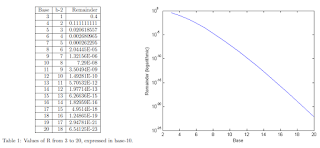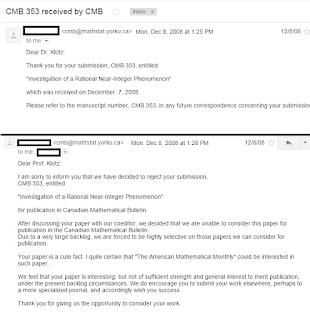## Saturday, 26 September 2015

### My first discovery: Pandigital Slinky Ratios

If you plug the quotient 987654321 / 123456789 into a calculator, you will find it is very close to 8. It is approximately 8.000000072900... This seems like a coincidence, but it isn't! Figuring out the pattern to these numbers was the first thing I ever "discovered," with the help of my uncle. I tried to publish my proof, and was rejected in three minutes.The slinky is a metaphor.

My father actually showed me this trick on an old calculator when I was about six years old. I just thought he was trying to show that two big numbers make a small number; I didn't really understand what division was at the time. I spent a lot of time in highschool playing with my calculator. To pass the time I would button-mash random four-digit numbers and try to divide them by prime factors until I got to a prime number; I generally liked playing with calculators.

I do not remember the historical sequence of events that lead to my discovery, but I always curious about why this was so close to 8. On the Windows calculator, you could enter calculations in base-8, base-16, and binary as well as decimal. So, one day I tried doing the same thing in those modes. If you do the same type of calculation in hexadecimal, FEDCBA987654321/123456789ABCDEF, you get E.0000000001D46... and the remainder is smaller than in base-10 (there are nine zeros there instead of seven). In base 8, 7654321/1234567 gets you 6.00000527, only five zeros. So, a pattern emerged: the bigger the counting base, the closer the slinky ratio gets to the second biggest digit. Around 2005, in first or second year of university, I downloaded a calculator app (we used to call them programs!) that would go up to base 36 (using 0-9 and A-Z). This was the highest I could test, and the pattern continued.

I wanted to figure out why.

Writing this blog post and searching my email for "987654321" I found a few awkward attempts to email math professors asking for help proving this. In my training as a physicist, I learned that one of the best ways to solve a math problem is to get a computer to do it, and a program like Maple or Mathematica can figure this out after like 30 seconds of typing and pressing Enter. However, there is a more interesting way to prove this relationship. The main insight came with the help of my uncle Bob, who was a mathematician before becoming a lawyer, when I asked him about the problem. The Bob Proof is more illuminating than a computer algebra output, so I'll discuss that.

In order to figure out how this trend generalizes, the first step is to write down what the operation actually is. So, for some counting base b, the numerator is:

$\sum_{n=1}^{b-1}\left(b-n\right)b^{b-n-1}$

And the denominator is:

$\sum_{n=1}^{b-1}nb^{b-n-1}$

So we divide them and observe empirically:

$\frac{\sum_{n=1}^{b-1}\left(b-n\right)b^{b-n-1}}{\sum_{n=1}^{b-1}nb^{b-n-1}} \approx b-2 + \epsilon$

And we want to know what gives*.

Bob's insight was to rewrite each expression as a sum of oneful numbers. For the denominator, the term can be expressed as 1+11+111 etc. Do this to some value of ones, and you'll get a number that looks like 123456... because each iteration increases the final digit. You can also express it as (1+(10+1)+(100+10+1)...) with the appropriate definition of 10. It is also the case that in any base**, that a oneful number times the largest digit is one less than a power (e.g. 111x9=999=1000-1).

So, we can rewrite the oneful denominator as a chain of oneful sequences:

$\sum_{n=1}^{b-1}nb^{b-n-1}=\frac{b-1}{b-1}+\frac{b^2-1}{b-1}+\frac{b^3-1}{b-1}...$

And now factor:

$\sum_{n=1}^{b-1}nb^{b-n-1}=\frac{\sum_{n=1}^{b-1}b^{n}}{b-1}-b-1$

Now, there is a trick involved that I don't quite remember. I might have just gotten a computer to figure it out, cheapening my whole example of why this proof is more insightful than just using computer algebra, but anyway it relies on the identity:

$\sum_{n=1}^{x-1}x^{x}=\frac{x^x}{x-1}-\frac{x}{x-1}$

I honestly cannot remember why this is true, and I am failing to prove it by induction. So to prevent this whole post from falling apart, proof of this particular identity will be left as an exercise for the reader. Anyway, using this identity in the previous expression, we get a sigmaless term for the denominator:

$\sum_{n=1}^{b-1}nb^{b-n-1}=\frac{b^{b}-1}{(b-1)^2}-1$

There is a similar procedure for the numerator. Instead of 1+11+111...we add terms of equal magnitude, starting with 111111...11111 and going to 1000...0000, replacing a 1 with a zero in each term. So in base-6, we have 10000+11000+11100+11110+11111=54321. Keep adding these, and if you don't exceed the number of terms required for your base, you'll get your descending slinky.

To simplify the numerator with this method, the logic is similar for the denominator. In short:

$\sum_{n=1}^{b-1}\left(b-n\right)b^{b-n-1}=\sum_{n=1}^{b-1}\frac{\left(b^{b-n}-1\right)b^{n-1}}{b-1}=b^{b-1}-\frac{b^{b}-b}{b\left(b-1\right)^{2}}$

So now we have expressions for the numerator, the descending slinky number ending in ...54321, and the denominator, the ascending slinky starting with 12345...., and both are in "closed form." So, we can divide them and simplify, and we get:
$\frac{\sum_{n=1}^{b-1}\left(b-n\right)b^{b-n-1}}{\sum_{n=1}^{b-1}nb^{b-n-1}}=\left(b-2\right)+\frac{\left(b-1\right)^{3}}{b^{b}-b^{2}+b-1}$

And that is our result! Just looking at it, we see that it is (b-2) plus some stuff, and the stuff has a $b^{b}$ on the bottom and a $b^{3}$ on top, so it will be very small if b gets much bigger than 3. But there's more we can learn from this.

In base-10, the value we get starts with 8.0000000729. You may recognize those last three digits as the cube of 9.  If you take only the largest term in the denominator of the remainder, $b^b$, you will find that the first bit of the remainder is $(b-1)^{3}\cdot b^{-b}$, which is $9^3\cdot 10^{-10}$, which is what we see. This was before I knew about or understood perturbation theory, so nowadays I would call this the next-to-leading order term.That remainder gets real small real fast.

Like I said, a computer can easily figure this out. Bob and I may have been the first people to do this, but weren't the last. In 2013 the question was asked on Math Stack Exchange, and a user WimC gave a similar answer, with an expression in terms of a sum of diminishing terms.

Now, I am much better at finding literature online, so I will do a retroactive literature review. A solution may have been found in 2004 but I didn't know at the time. In fact, this was discussed in 1987, the year of my birth, where P.R. Pujado shows that the eight closeness is not a coincidence, but the result of a pattern in a growing series. The generalized b-base form is not presented there.

In late 2008**, I was working as a post-bach researcher at Sunnybrook hospital studying bubbles, and decided to write up my findings and get them published; my first attempt to do so. I wrote a paper summarizing what I have explained here. Besides lab reports, my only piece of scientific writing was my undergraduate thesis on general relativity, and I still hadn't learned how to write like a grown-up. I sent it off the the Canadian Mathematical Bulletin. On December 8, 2008, at 1:25 PM I received an email saying that the editors were reviewing my paper, and it could take about six months. At 1:28 PM, I received another email saying that it had been rejected. Their reasoning? "Your paper is a cute fact." Well, they weren't wrong. They suggested I send it to the American Mathematical Monthly, which also rejected it, and then I sent it off by paper mail to the Journal of Recreational Mathematics, who never got back to me, and that was the end of that. It is perfectly reasonable that journals would reject this and I don't hold it against them.In those three minutes, I was upgraded from Dr. to Prof.

This problem, the process of solving it, and my failure to get the solution published, ignited a passion in me that made me want to become a published mathematician. I generally find physics more interesting than mathematics, but there's a certain purity to coming up with and proving a new mathematical fact, and I wanted to have one of my own. I eventually did, about five years later, and this may be what indirectly set me on that path.

P.S. If someone can leave a comment explaining how to centre the LaTeX, I would appreciate.

*$\epsilon$ is math notation for something very small
**no rhyme intended